# AP Statistics : How to simulate random behavior

## Example Questions

### Example Question #1 : Random Variables

Which of the following is NOT a discrete random variable?

Number of lip products in a girl's makeup bag

Amount of money in a savings account

Number of midterms taken in a 10-week course

All of these answers are discrete variables

Time taken to watch the first 4 seasons a TV show

Time taken to watch the first 4 seasons a TV show

Explanation:

By definition, a discrete random variable is a random variable whose values can be "counted" one by one. A continuous random variable is a random variable that can take any value on a certain interval. Of these choices, the number of lip products, the amount of money, and the number of midterms taken are all discrete random variables, as the respective values can be counted; however, the time taken to watch the first four seasons of a TV show is a continuous random variable, as not everyone will take the same amount of time to watch all those episodes (i.e. some might fastf-orward/replay parts of episodes).

### Example Question #2 : Random Variables

Police estimate that 85% of drivers do not text and drive. They set up a safety roadblock at a busy intersection to check for this infraction.

What is the probability that the first texter is in the thirteenth car stopped?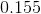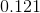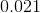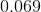Explanation:

This question pertains to the concept of Geometric Probability Distribution, which states that the probability oftrials until the first success is as follows: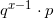In this equation,is the probability of success (in this case, a driver caught texting), and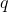is the probability of failure.

In this particular problem,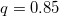, and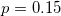. To calculate the probability of finding that the first driver caught texting is in the thirteenth car stopped, we can calculate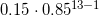. The final answer is.